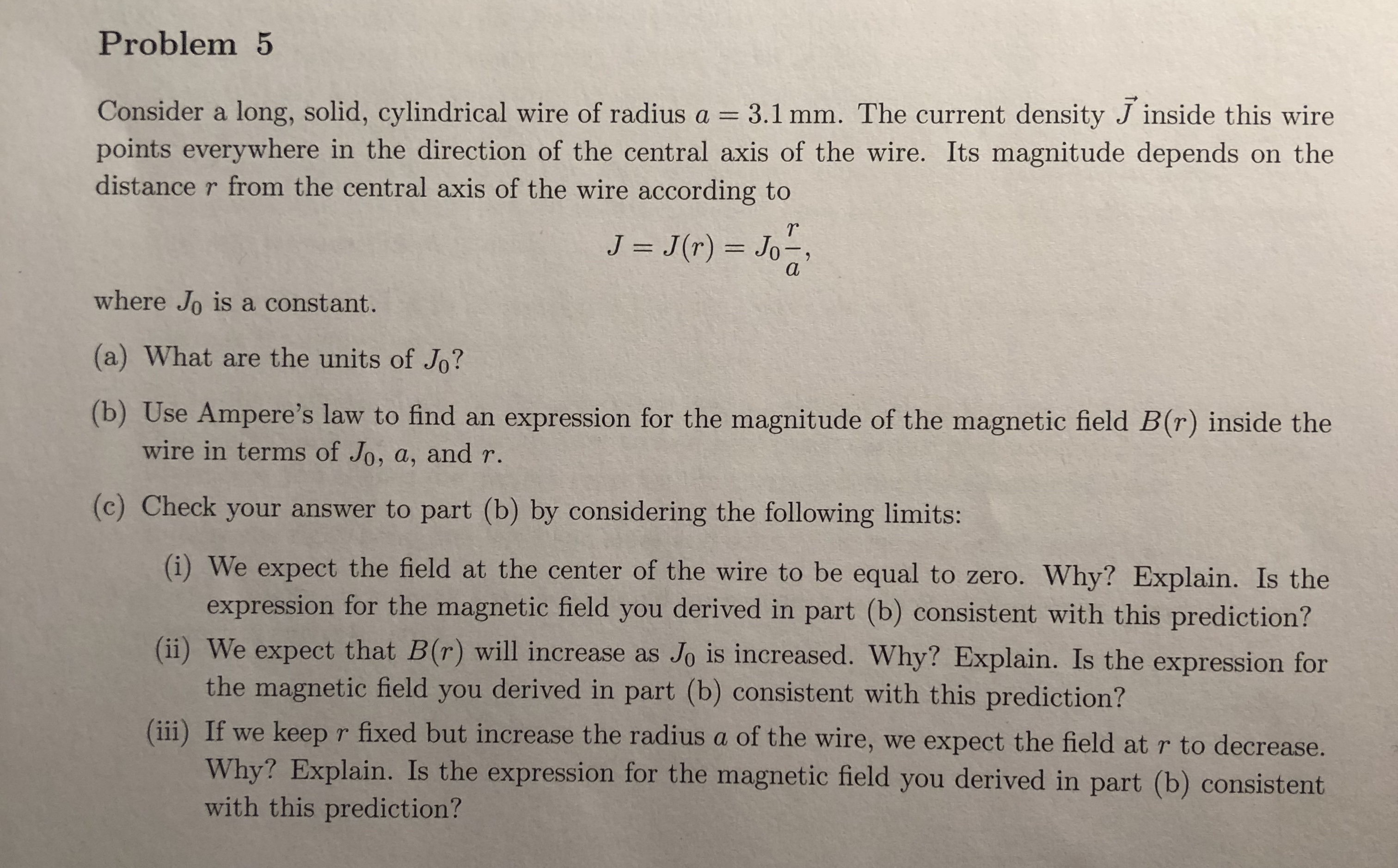# Problem5Consider a long, solid, cylindrical wire of radius a 3.1 mm. The current density J inside this wirepoints everywhere in the direction of the central axis of the wire. Its magnitude depends on thedistance r from the central axis of the wire according toTrwhere Jo is a constant.(a) What are the units of Jo?(b) Use Ampere's law to find an expression for the magnitude of the magnetic field B(r) inside thewire in terms of Jo, a, and r(c) Check your answer to part (b) by considering the following limits:(i) We expect the field at the center of the wire to be equal to zero. Why? Explain. Is theexpression for the magnetic field you derived in part (b) consistent with this prediction?(i) We expect that B(r) will increase as Jo is increased. Why? Explain. Is the expression forthe magnetic field you derived in part (b) consistent with this prediction?(ii) If we keep r fixed but increase the radius a of the wire, we expect the field at r to decrease.Why? Explain. Is the expression for the magnetic field you derived in part (b) consistentwith this prediction?

Questionhelp_outlineImage TranscriptioncloseProblem5 Consider a long, solid, cylindrical wire of radius a 3.1 mm. The current density J inside this wire points everywhere in the direction of the central axis of the wire. Its magnitude depends on the distance r from the central axis of the wire according to Tr where Jo is a constant. (a) What are the units of Jo? (b) Use Ampere's law to find an expression for the magnitude of the magnetic field B(r) inside the wire in terms of Jo, a, and r (c) Check your answer to part (b) by considering the following limits: (i) We expect the field at the center of the wire to be equal to zero. Why? Explain. Is the expression for the magnetic field you derived in part (b) consistent with this prediction? (i) We expect that B(r) will increase as Jo is increased. Why? Explain. Is the expression for the magnetic field you derived in part (b) consistent with this prediction? (ii) If we keep r fixed but increase the radius a of the wire, we expect the field at r to decrease. Why? Explain. Is the expression for the magnetic field you derived in part (b) consistent with this prediction? fullscreen
check_circleExpert Solution
Step 1

(a)

The expression for the current density is given as

Step 2

(b)

Ampere’s law ...

### Want to see the full answer?

See Solution

#### Want to see this answer and more?

Solutions are written by subject experts who are available 24/7. Questions are typically answered within 1 hour*

See Solution
*Response times may vary by subject and question
Tagged in

### Physics